﻿ 摆翼式波浪能发电海洋航行器无水实验系统
 舰船科学技术2020, Vol. 42Issue (2): 72-76PDF

1. 武汉理工大学 能源与动力工程学院，湖北 武汉 430063;
2. 国家水运安全工程技术研究中心 可靠性工程研究所，湖北 武汉 430063

Research on waterless testing system for the flapping-wings wave-powered AUV
WANG Yu-gong1,2, ZHAO Jiang-bin1,2, ZHU Feng-shen1,2, ZHOU Jian-lin1,2
1. School of Energy and Power Engineering, Wuhan University of Technology, Wuhan 430063, China;
2. Reliability Engineering Institute, National Engineering Research Center for Water Transport Safety (WTS), Wuhan 430063, China
Abstract: For testing the power generation capacity of the flapping-wings wave-powered AUV, a scheme of waterless testing system which can simulate the coupling action between waves and the vehicle under the actual sea condition is proposed. By studying the principle of AUV power generation, establishing kinematics model, studying the relationship between model parameters and practice, and combining the control and data acquisition technology, the design and construction of the waterless testing system were completed. Through CFD simulation and test data comparison, the results show that the testing system can effectively simulate wave load for the wing, which proves the correctness and reliability of the system.
Key words: wave simulation     waterless experiment     wave-powered     numerical simulation
0 引　言

AUV（Autonomous Underwater Vehicle）等小型海洋航行器作为海洋中的移动平台在与海洋相关的诸多领域有着极为广阔的应用前景，但其动力源主要依靠航行器自身携带的蓄电池，续航力低。在现有的新能源解决方案中，波浪能不受时空限制，潜力巨大，已经有船舶利用波浪发电的方案。传统研究将波浪能转换装置内置于航行器壳体中，依靠惯性发电，产生电能较少[1,3]。有一种新的摆翼式波浪能发电小型海洋航行器的研究方案，通过航行器两侧摆翼振动，使航行器在海洋波面运动中发电，相较内置式发电能力更强。设计对波浪能发电航行器实况海测前的模拟实验能够降低成本并提高开发效率。如何模拟真实海况下的运动十分重要。

1 工作原理 1.1 波浪能发电航行器模型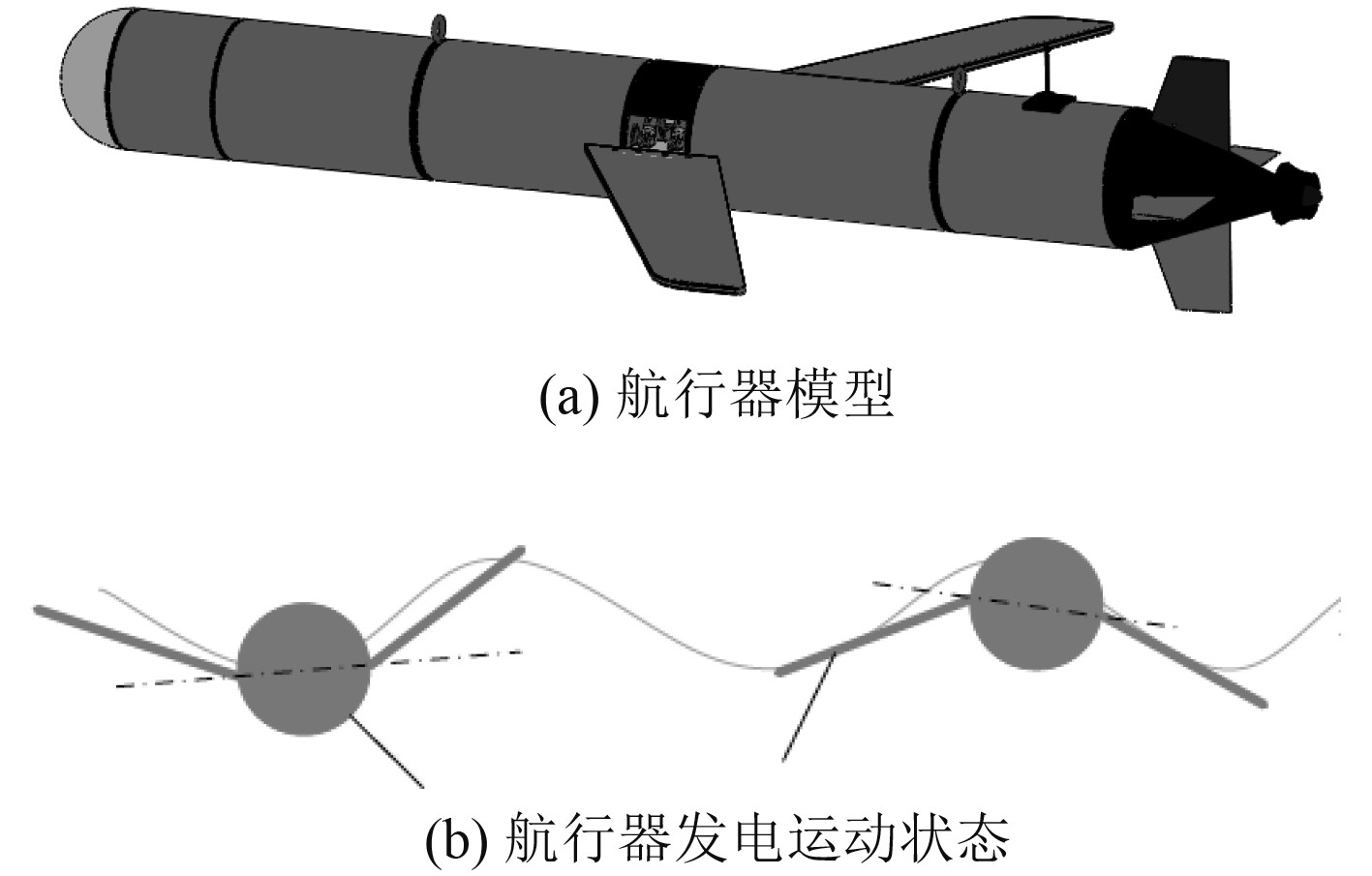图 1 摆翼式波浪能发电海洋航行器 Fig. 1 The flapping-wings wave-powered AUV
1.2 运动学模型

 $f(x,t) = \frac{H}{2}\cos (kx - \omega t) {\text{。}}$ (1)

 ${u_x} = \frac{{{\text{π}} H}}{T}\frac{{ch[k(z + d)]}}{{sh(kh)}}\cos (kx - \omega t){\text{，}}$ (2)
 ${u_z} = \frac{{{\text{π}} H}}{T}\frac{{sh[k(z + d)]}}{{sh(kh)}}\sin (kx - \omega t){\text{。}}$ (3)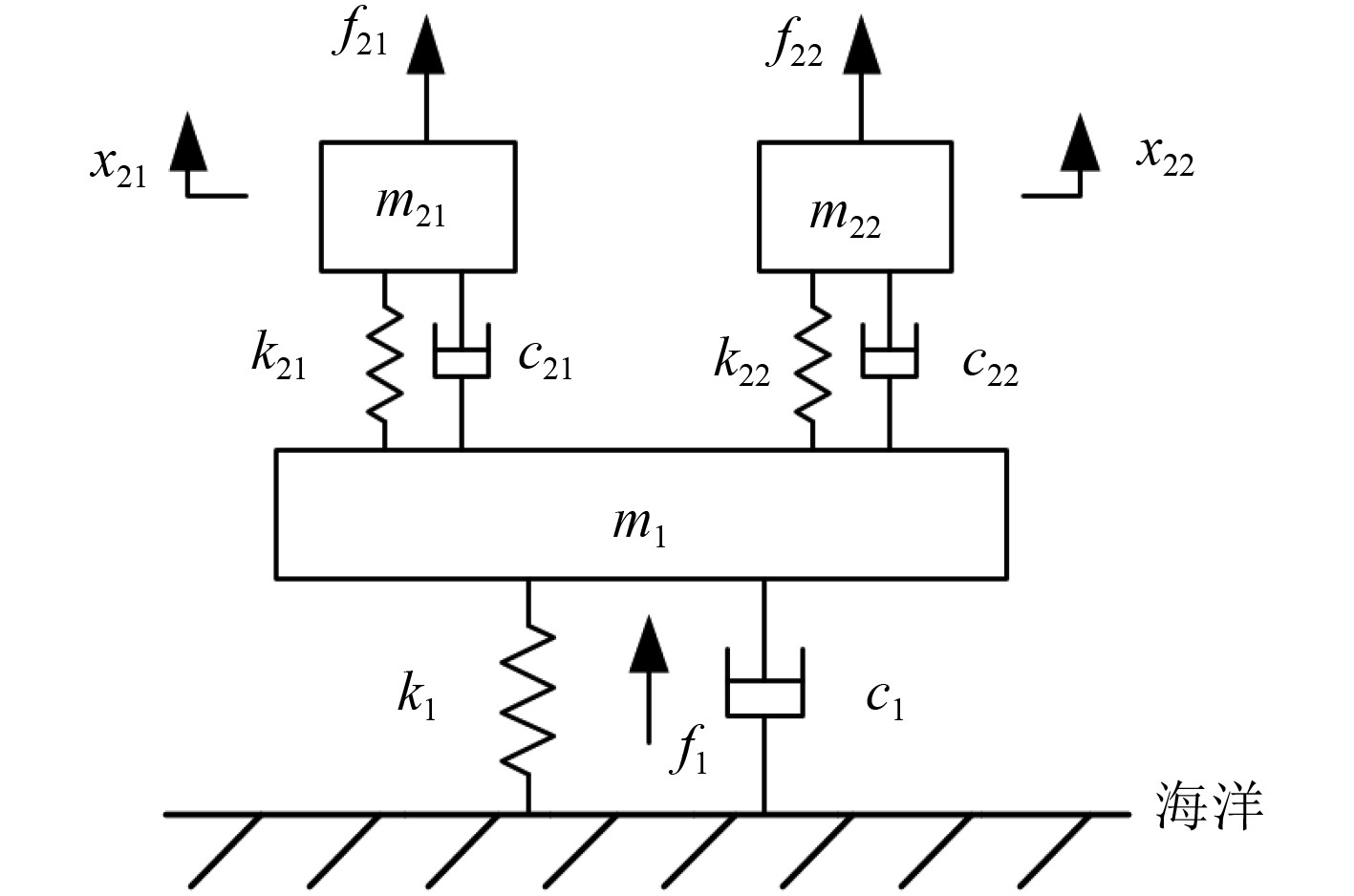图 2 航行器振动模型 Fig. 2 Vehicle vibration model

1.3 系统原理与设计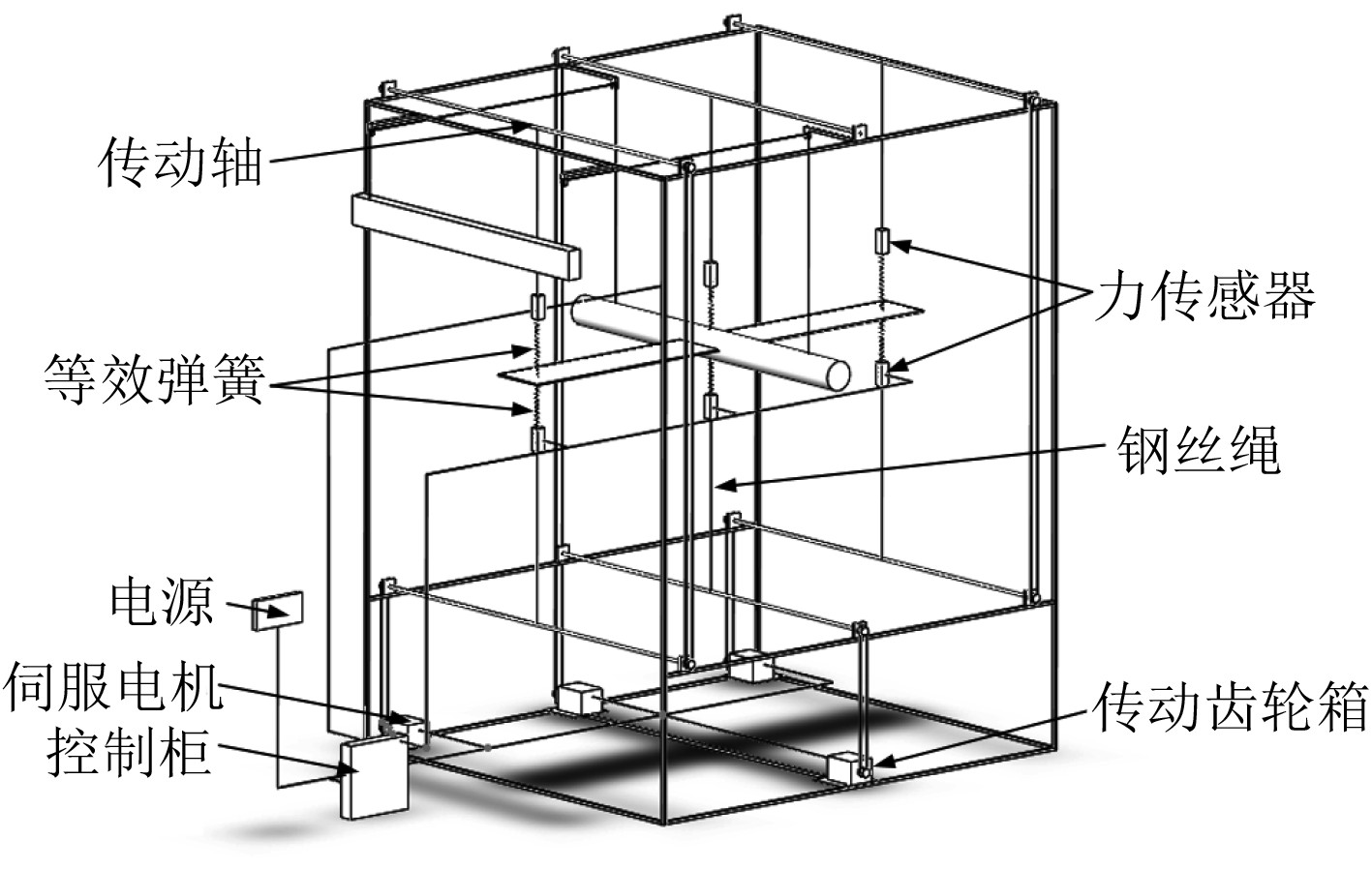图 3 实验系统台架设计 Fig. 3 The design of testing system bench

 $\Delta \varphi = \frac{{L\Delta x}}{{2{\text{π}} }}{\text{。}}$ (4)

 $\left\{ \begin{array}{l} {k_1} = \rho gA {\text{，}}\\ {k_{21}} = {k_{22}} = {{{k_t}} / {\Delta x}} {\text{，}} \\ {c_{21}} = {c_{22}} = {{Cn} / {\Delta x}}{\text{。}} \end{array} \right.$ (5)

${k_2} = {k_{21}} = {k_{22}}$ ${c_2} = {c_{21}} = {c_{22}}$ ，通过Lagrange方程建立运动数学模型为：

 $\begin{split} &\left[ {\begin{array}{*{20}{c}} {{m_1}}&0&0 \\ 0&{{m_{21}}}&0 \\ 0&0&{{m_{22}}} \end{array}} \right]\left[ {\begin{array}{*{20}{c}} {{{\ddot x}_1}} \\ {{{\ddot x}_{21}}} \\ {{{\ddot x}_{22}}} \end{array}} \right] + \left[ {\begin{array}{*{20}{c}} {{c_1} + 2{c_2}}&{ - {c_2}}&{ - {c_2}} \\ { - {c_2}}&{{c_2}}&0 \\ { - {c_2}}&0&{{c_2}} \end{array}} \right]\times\\ &\left[ {\begin{array}{*{20}{c}} {{{\dot x}_1}} \\ {{{\dot x}_{21}}} \\ {{{\dot x}_{22}}} \end{array}} \right] + \left[ {\begin{array}{*{20}{c}} {{k_1} + 2{k_2}}&{ - {k_2}}&{ - {k_2}} \\ { - {k_2}}&{{k_2}}&0 \\ { - {k_2}}&0&{{k_2}} \end{array}} \right]\left[ {\begin{array}{*{20}{c}} {{x_1}} \\ {{x_{21}}} \\ {{x_{22}}} \end{array}} \right] = \left[ {\begin{array}{*{20}{c}} {{f_1}} \\ {{f_{21}}} \\ {{f_{22}}} \end{array}} \right]{\text{。}} \end{split}$ (6)

 $\begin{split} & p = {c_2}{({\dot x_{21}} - {\dot x_1})^2} =\\ & {c_2}{\omega ^2}{\left[ {{X_{21}}\cos (\omega t) - {X_1}\cos (\omega t + \Delta \varphi )} \right]^2} {\text{，}} \end{split}$ (7)
 ${p_a} = \frac{2}{T}\int_0^T {p(t){\rm d}t} = {c_2}{\omega ^2}\left[ {{X_1}^2 + {X_{21}}^2 - 2{X_1}{X_{21}}\cos \Delta \varphi } \right]{\text{。}}$ (8)
2 功能实现与实物模型 2.1 系统功能关键技术

1）波浪模拟

 \left\{ \begin{aligned} & r = \frac{{SH}}{{2P}} {\text{，}} \\ & v = \frac{{SHR\omega }}{{2P}}{\text{。}} \end{aligned} \right. (9)

2）数据采集

2.2 上位机系统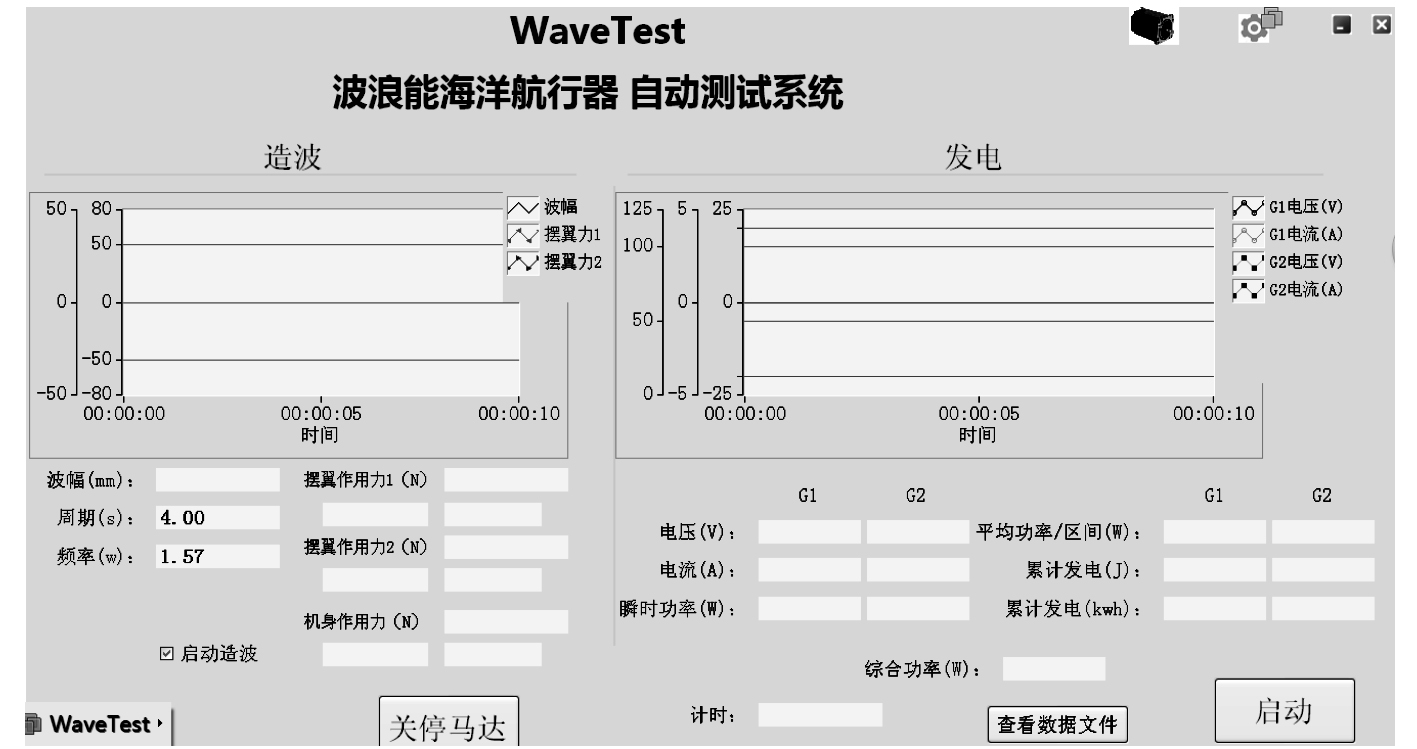图 4 系统界面 Fig. 4 The system interface

2.3 实物结构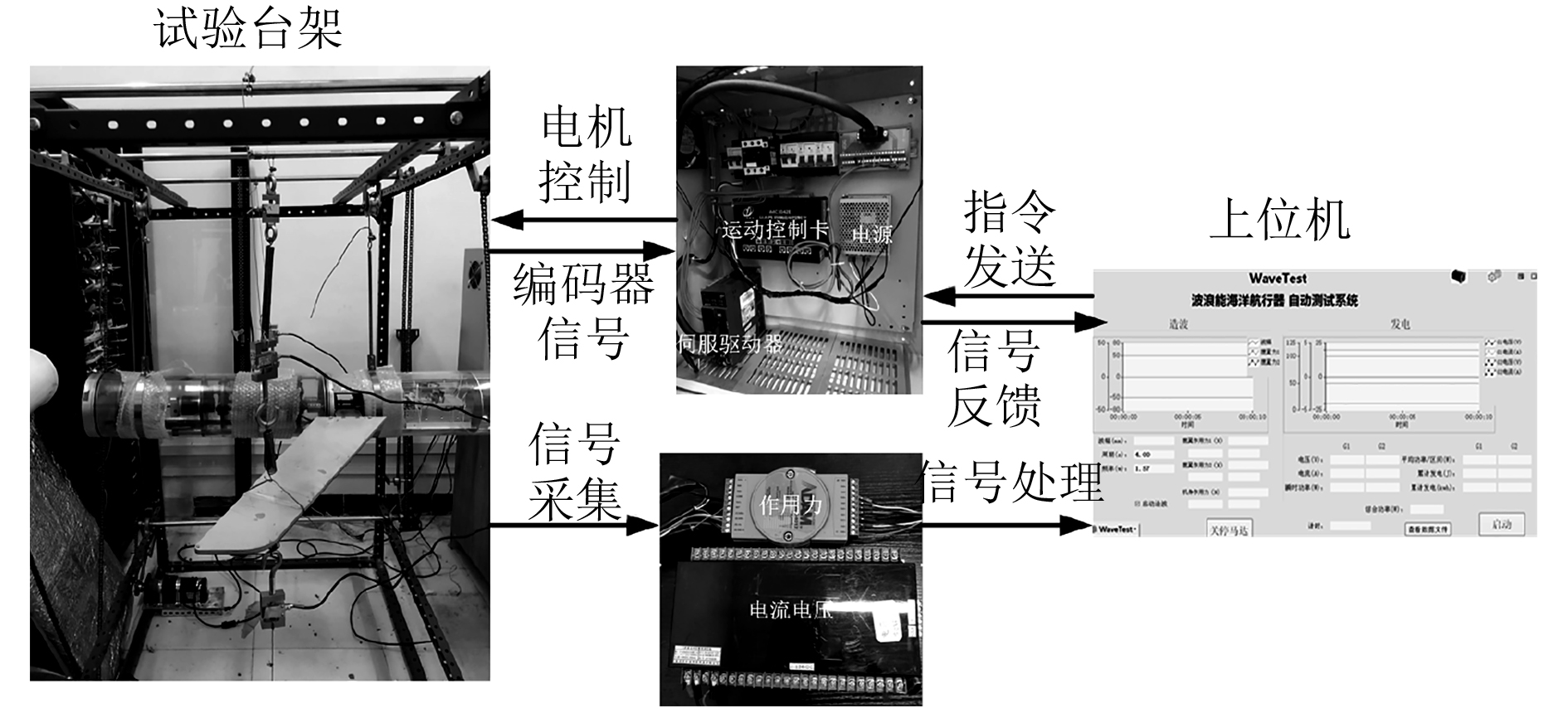图 5 实验系统结构 Fig. 5 The structure of testing system

3 实验验证 3.1 CFD仿真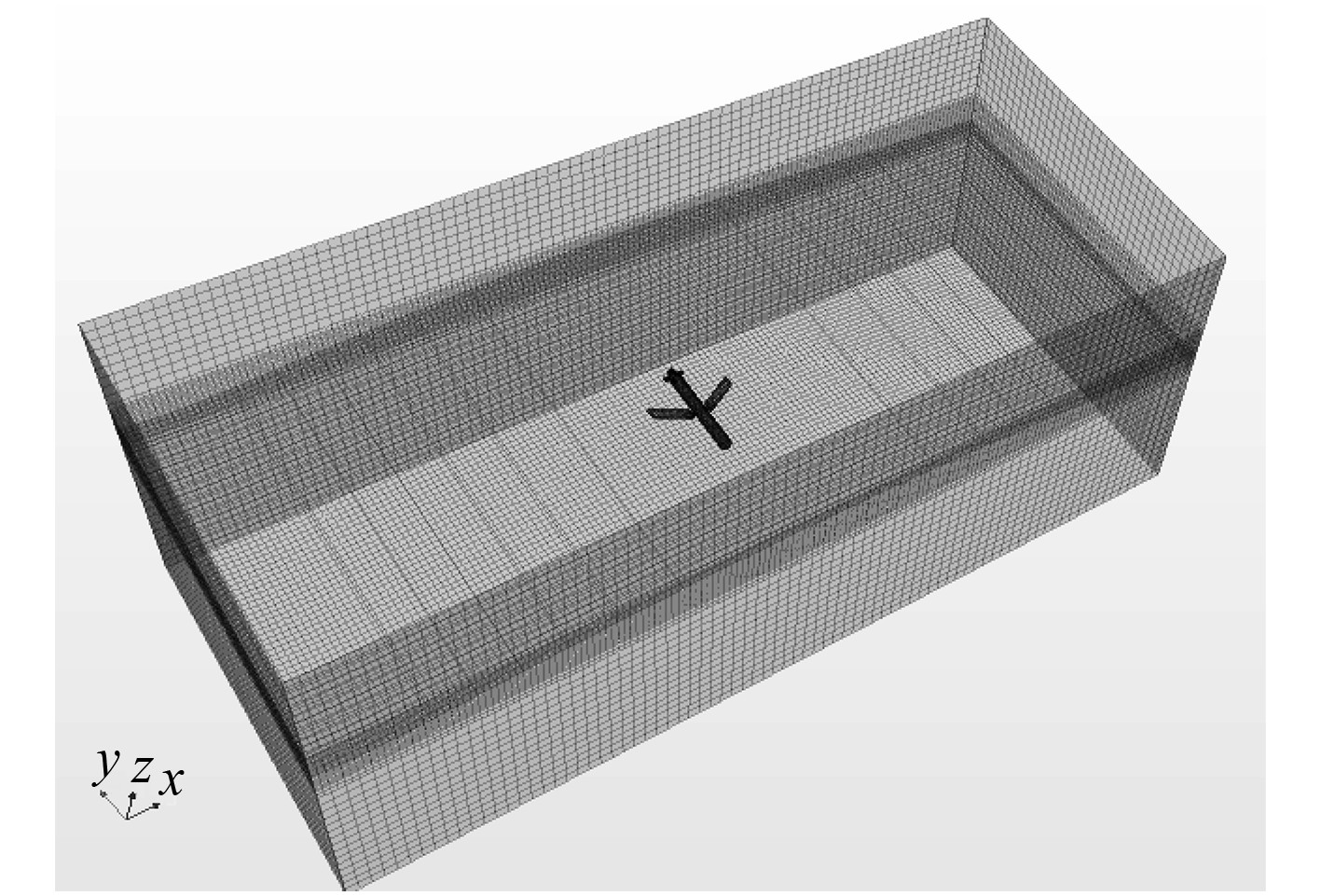图 6 计算域网格划分 Fig. 6 Meshing of Computational domain

3.2 无水实验表 1 实验系统参数 Tab.1 Testing system parameters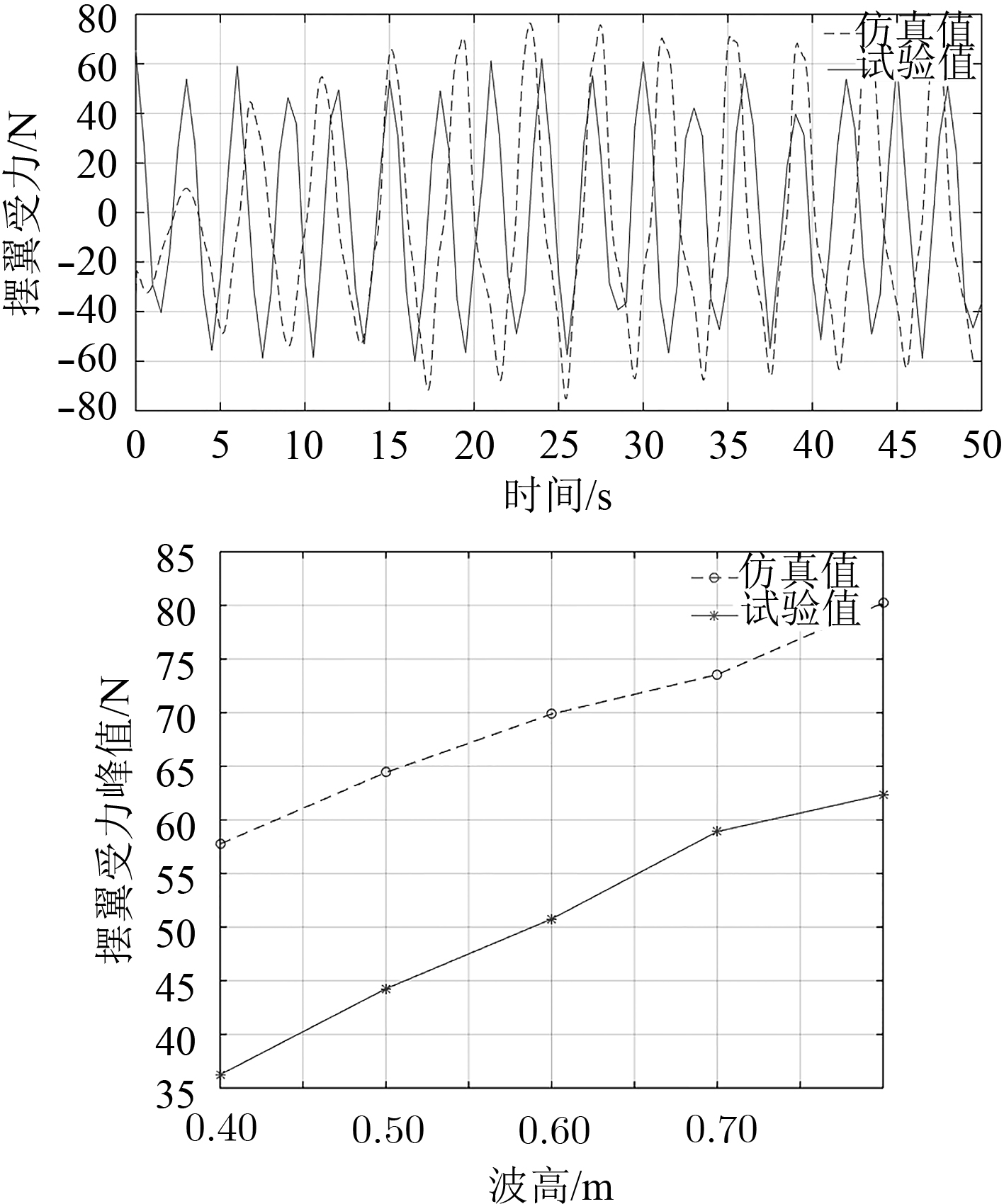图 7 仿真与实验结果对比 Fig. 7 Comparison of simulation and test results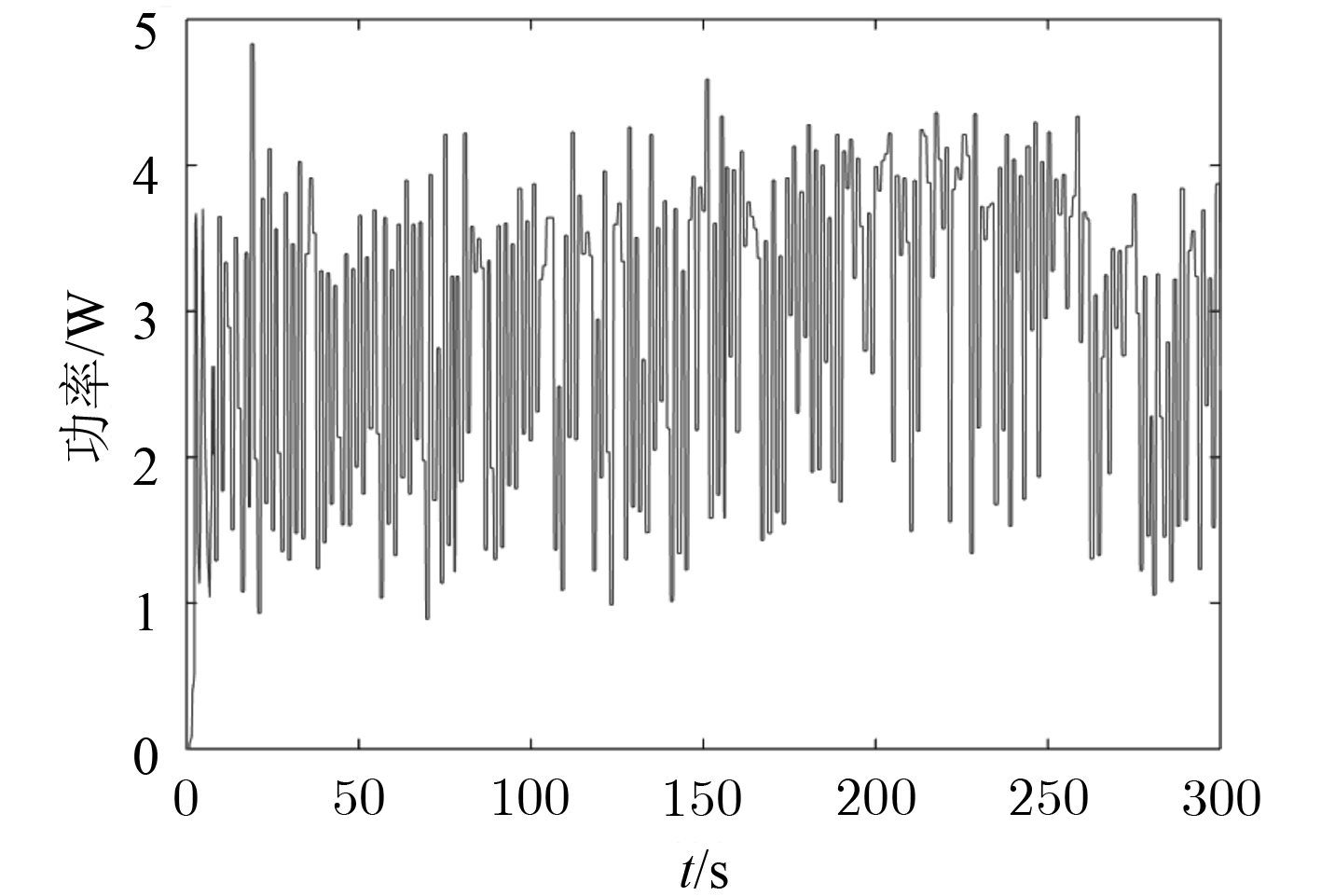图 8 波幅0.6 m时航行器实验输出功率 Fig. 8 The AUV test output power when the amplitude is 0.6 m
4 结　语

  顾煜炯, 谢典, 耿直. 波浪能发电技术研究进展[J]. 电网与清洁能源, 2016, 32(5): 83-87. DOI:10.3969/j.issn.1674-3814.2016.05.015  周广钰. 船舶上可装载的波浪能发电装置设计[J]. 舰船科学技术, 2015, 37(7): 216-220. DOI:10.3404/j.issn.1672-7649.2015.07.051  RHINEFRANK K, AGAMLOH E B, VON JOUNANE A, et al. Novel ocean energy permanent magnet linear generator buoy[J]. Renewable Energy, 2006, 31(9): 1279-1298. DOI:10.1016/j.renene.2005.07.005  DREW B, PLUMMER A R, SAHINKAYA M N. A review of wave energy converter technology[J]. Proceedings of the Institution of Mechanical Engineers, 2009, 223(A8).  张广辉. 主动吸收式水槽造波机控制系统设计[D]. 大连: 大连理工大学, 2017.  程少科. 造波系统能量优化研究及其机构设计[D]. 太原: 太原科技大学, 2016.  杨绍辉, 何宏舟, 曲泉铀. 多点直驱式波浪能发电系统仿真分析与试验研究[J]. 太阳能学报, 2014, 35(8): 1375-1380. DOI:10.3969/j.issn.0254-0096.2014.08.010  齐家龙. 带波浪模拟的浮力摆式波浪能发电装置的试验研究[D]. 杭州: 浙江大学, 2014.  俞科云. 波浪运动模拟装置设计[J]. 中国水运(下半月), 2014, 14(8): 150-151+153.  Ruud K, Frank N. Wave energy technology brief[R]. Masdar City: IRENA Headquarters, 2014.  张佳, 窦丽华, 白永强. 伺服电机测试实验平台设计与实现[J]. 实验技术与管理, 2011, 28(6): 68-73. DOI:10.3969/j.issn.1002-4956.2011.06.019  沈凤梅. 基于Modbus协议的实时电流监控系统开发[J]. 制造业自动化, 2018, 40(5): 36-37+81. DOI:10.3969/j.issn.1009-0134.2018.05.009  阮波, 陈永军. 基于C#的无刷直流电动机控制系统综合实验平台开发[J]. 实验技术与管理, 2016, 33(5): 99-104.